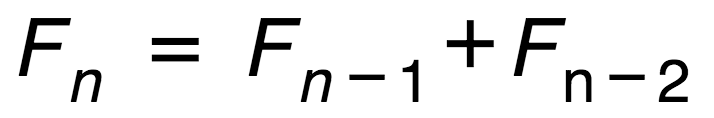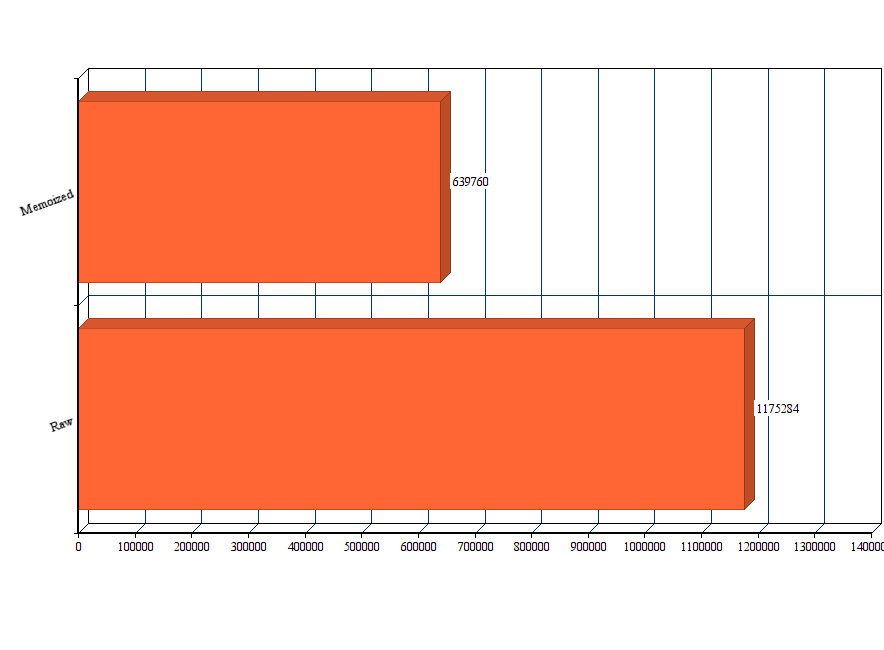06月12, 2016

# 【译】我从用 JavaScript 写斐波那契生成器中学到的令人惊讶的 7 件事

#### 采用新特性

• R Rest 和 Spread
• O 对象字面量简写 (Object Literal Shortcuts)
• A 箭头函数 (Arrow Functions)
• D 解构和默认参数 (Destructuring & Default Parameters)
• M 模块 (Modules)
• AP 异步编程 (Asynchronous Programming: promises & generators)

#### 斐波那契数列是什么？0, 1, 1, 2, 3, 5, 8, 13, 21, 34…

#### 生成器函数是什么？

``````{
value: Any,
done: Boolean
}
``````

`value` 属性包含一个被 yield 的值，`done` 标志表明生成器是否已经 yield 到了最后一个值。

### 1. 生成器与递归不同``````function* fib (n, current = 0, next = 1) {
if (n === 0) {
return 0;
}

yield current;
yield* fib(n - 1, next, current + next);
}
``````

`if` 条件当 `n` 为 0 时，通过使用 `return` 代替 `yield` 让循环终止。如果你不传 `n`，它的值为 `undefined`，于是 `n - 1` 变成 `NaN`，结果是生成器函数将永远不会终止。

(ಥ﹏ಥ)

7. Let tailCall be IsInTailPosition(thisCall).

8. Return EvaluateDirectCall(func, thisValue, Arguments, tailCall).

`IsInTailPosition` 对生成器返回 false。 (看 14.6.1):

5. If body is the FunctionBody of a GeneratorBody, return false.

``````function* fib (n) {
const isInfinite = n === undefined;
let current = 0;
let next = 1;

while (isInfinite || n--) {
yield current;
[current, next] = [next, current + next];
}
}
``````

### 2. 使用参数限制迭代次数

``````const [...arr] = generator(8);
``````

┻━┻ ︵ヽ(`Д´)ﾉ︵ ┻━┻

┬─┬ ノ( ゜-゜ノ)

### 3. 注意使用缓存函数（Memoized Function）

#### 什么是缓存函数？

``````const memo = [];

const fib = (n) => {
if (memo[n] !== undefined) return memo[n];

let current = 0;
let next = 1;

for (let i = 0; i < n; i++) {
memo[i] = current;
[current, next] = [next, current + next];
}

return current;
};

function* gen (n = 79) {
fib(n);
//此处应该是  yield* memo.slice(0, n)？——译者注
yield* memo.slice(0, n + 1);
}

export default gen;
``````

#### 修改：

`yield*``yield` 的一个特殊形式，它可以代理给另一个生成器或者可迭代对象。例如：

``````const a = [1, 2, 3];
const b = [4, 5, 6];

function* c () {
yield 7;
yield 8;
yield 9;
}

function* gen () {
yield* a;
yield* b;
yield* c();
yield 10;
}

const [...sequence] = gen();
console.log(sequence); // [1,2,3,4,5,6,7,8,9,10]
``````

#### 性能测试 (Benchmarks)### 4. JavaScript 需要一个内置的 API 来精确计时

``````const nsTime = (hrtime) => hrtime * 1e9 + hrtime;
``````

``````import iterativefib from "iterativefib";
import memofib from "memofib";
import range from "test/helpers/range";

const nsTime = (hrtime) => hrtime * 1e9 + hrtime;

const profile = () => {
const numbers = 79;
const msg = `Profile with \${ numbers } numbers`;

const fibGen = iterativefib();
const fibStart = process.hrtime();
range(1, numbers).map(() => fibGen.next().value);
const fibDiff = process.hrtime(fibStart);

const memoGen = memofib();
const memoStart = process.hrtime();
range(1, numbers).map(() => memoGen.next().value);
const memoDiff = process.hrtime(memoStart);

const original = nsTime(fibDiff);
const memoized = nsTime(memoDiff);

console.log(msg);
console.log(`
original: \${ original }ns
memoized: \${ memoized }ns
`);
};

profile();
``````

`hrtime()` 里我最喜欢的特性是你可以传递开始时间进去获得从开始时间到当前经过的时间段——这正是性能测试所需要的。

### 5. 当心浮点数精度问题

``````const sqrt = Math.sqrt;
const pow = Math.pow;

const fibCalc = n => Math.round(
(1 / sqrt(5)) *
(
pow(((1 + sqrt(5)) / 2), n) -
pow(((1 - sqrt(5)) / 2), n)
)
);
``````

``````const sqrt = Math.sqrt;
const pow = Math.pow;

const fibCalc = n => Math.round(
(1 / sqrt(5)) *
(
pow(((1 + sqrt(5)) / 2), n) -
pow(((1 - sqrt(5)) / 2), n)
)
);

function* fib (n) {
const isInfinite = n === undefined;
let current = 0;

while (isInfinite || n--) {
yield fibCalc(current);
current++;
}
}
``````

``````Profile with 79 numbers
original: 901643ns
memoized: 544423ns
formula:  311068ns
``````

¯(º_o)/¯

### 6. 了解你的极限

• 我不知道使用标准的 JavaScript `Number` 类型可以从这个数列中获得多少精确值。
• 我不知道从公式版的实现中可以获得多少精确值。
• 我不知道需要多少层递归调用来获得这些精确值。

``````const lookup = [0, 1, 1, 2, 3, 5, 8, 13, 21, 34, 55, 89, 144, 233, 377, 610,
987, 1597, 2584, 4181, 6765, 10946, 17711, 28657, 46368, 75025, 121393,
196418, 317811, 514229, 832040, 1346269, 2178309, 3524578, 5702887, 9227465,
14930352, 24157817, 39088169, 63245986, 102334155, 165580141, 267914296,
433494437, 701408733, 1134903170, 1836311903, 2971215073, 4807526976,
7778742049, 12586269025, 20365011074, 32951280099, 53316291173, 86267571272,
139583862445, 225851433717, 365435296162, 591286729879, 956722026041,
1548008755920, 2504730781961, 4052739537881, 6557470319842, 10610209857723,
17167680177565, 27777890035288, 44945570212853, 72723460248141,
117669030460994, 190392490709135, 308061521170129, 498454011879264,
806515533049393, 1304969544928657, 2111485077978050, 3416454622906707,
5527939700884757, 8944394323791464];

function* fib (n = 79) {
if (n > 79) throw new Error("Values are not available for n > 79.");
yield* lookup.slice(0, n);
}
``````

``````Profile with 79 numbers
original: 890088ns
memoized: 366415ns
formula:  309792ns
lookup:   191683ns
``````

``````const memo = [0, 1];

const fib = (n) =>
memo[n] !== undefined ? memo[n] :
memo[n] = fib(n - 1) + fib(n - 2);

function* gen (n = 79) {
if (n > 79) throw new Error("Accurate values are not available for n > 79.");
fib(n);
yield* memo.slice(0, n);
}

export default gen;
``````

#### 注意极限

• 如果你使用浮点数学公式，你应该要明确测试其精度范围。
• 如果你使用一个数列，这个数列以指数方式增长，你需要算出在 JS 的 `Number` 类型限制下你可以生成多少个数值。
• 如果你的极限足够小，考虑预生成一个查找表来加速你的应用。

### 7. 许多对象如生成器一样

``````let arr = [1,2,3];
let foo = arr[Symbol.iterator]();

arr.forEach(() => console.log( foo.next() ));
console.log(foo.next());
// { value: 1, done: false }
// { value: 2, done: false }
// { value: 3, done: false }
// { value: undefined, done: true }
``````

You can even build your own custom iterables:

``````const countToThree = {
a: 1,
b: 2,
c: 3
};

countToThree[Symbol.iterator] = function* () {
const keys = Object.keys(this);
const length = keys.length;

for (const key in this) {
yield this[key];
}
};

let [...three] = countToThree;
console.log(three); // [ 1, 2, 3 ]
``````

``````const abc = [1,2,3];

abc[Symbol.iterator] = function* () {
yield "a";
yield "b";
yield "c";
};

let [...output] = abc;
console.log(output);
abc.forEach(c => console.log(c));

// babel logs:
/*
[1,2,3]
1
2
3
*/

// Node logs:
/*
[ "a", "b", "c" ]
1
2
3
*/
``````

### 结论

1. 避免递归。生成器没有实现尾调用优化。
2. 允许参数限制你的生成器长度，这样你可以使用 ...rest 操作符来解构它们。
3. 缓存无限生成器会超出堆内存上线。
4. JavaScript 有两个完全不同的精确计时的 API。为什么不能统一起来？(ಥ﹏ಥ)
5. 浮点数精度问题会破坏基于公式的无限生成器。要当心。
6. 了解你的极限。你的生成器是否有足够的空间来满足你的应用需求？在这个空间里它获取的值是否足够精确？你是否将遇到你使用的数据类型的限制？JS 引擎是否有足够的内存来支持你的生成器运行直到你得到满意的结果？
7. 大部分内置对象的行为有点像生成器，通过可迭代协议，你可以定义你自己的可迭代对象。

-- EOF --